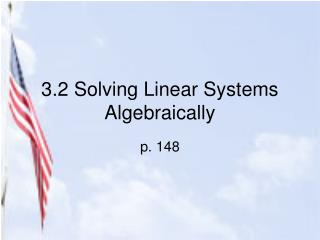DownloadDownload Presentation3.2 Solving Linear Systems Algebraically

# 3.2 Solving Linear Systems Algebraically

Download Presentation## 3.2 Solving Linear Systems Algebraically

- - - - - - - - - - - - - - - - - - - - - - - - - - - E N D - - - - - - - - - - - - - - - - - - - - - - - - - - -
##### Presentation Transcript

1. 2 Methods for Solving Algebraically • Substitution Method (used mostly when one of the equations has a variable with a coefficient of 1 or -1) • Linear Combination Method

2. Substitution Method • Solve one of the given equations for one of the variables. (whichever is the easiest to solve for) • Substitute the expression from step 1 into the other equation and solve for the remaining variable. • Substitute the value from step 2 into the revised equation from step 1 and solve for the 2nd variable. • Write the solution as an ordered pair (x,y).

3. 3x-y=13 2x+2y= -10 Solve the 1st eqn for y. 3x-y=13 -y= -3x+13 y=3x-13 Now substitute 3x-13 in for the y in the 2nd equation. 2x+2(3x-13)= -10 Now, solve for x. 2x+6x-26= -10 8x=16 x=2 Now substitute the 2 in for x in for the equation from step 1. y=3(2)-13 y=6-13 y=-7 Solution: (2,-7) Plug in to check soln. Ex: Solve using substitution method

4. Linear Combination Method • Multiply one or both equations by a real number so that when the equations are added together one variable will cancel out. • Add the 2 equations together. Solve for the remaining variable. • Substitute the value form step 2 into one of the original equations and solve for the other variable. • Write the solution as an ordered pair (x,y).

5. 2x-6y=19 -3x+2y=10 Multiply the entire 2nd eqn. by 3 so that the y’s will cancel. 2x-6y=19 -9x+6y=30 Now add the 2 equations. -7x=49 and solve for the variable. x=-7 Substitute the -7 in for x in one of the original equations. 2(-7)-6y=19 -14-6y=19 -6y=33 y= -11/2 Now write as an ordered pair. (-7, -11/2) Plug into both equations to check. Ex: Solve using lin. combo. method.

6. 9x-3y=15 -3x+y= -5 Which method? Substitution! Solve 2nd eqn for y. y=3x-5 9x-3(3x-5)=15 9x-9x+15=15 15=15 OK, so? What does this mean? Both equations are for the same line! ¸ many solutions Means any point on the line is a solution. Ex: Solve using either method.

7. 6x-4y=14 -3x+2y=7 Which method? Linear combo! Multiply 2nd eqn by 2. 6x-4y=14 -6x+4y=14 Add together. 0=28 Huh? What does this mean? It means the 2 lines are parallel. No solution Since the lines do not intersect, they have no points in common. Ex: Solve using either method.

8. Assignment